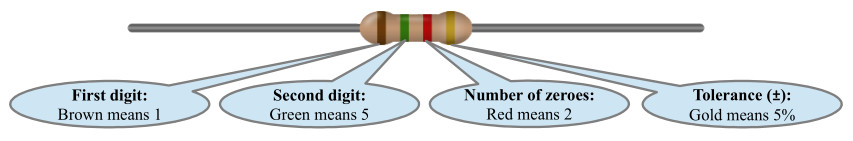Home » Handbook of O Level Physics » UNIT 24: INTRODUCTORY ELECTRONICS

# UNIT 24: INTRODUCTORY ELECTRONICS

• Thermionic emission: It is the effect in which when a metal is heated, some of the ‘free’ electrons gain enough kinetic energy to escape from the metal surface.

• Cathode-Ray Oscilloscope

• The component to be tested (e.g., a resistor or a mic) is connected across “Y-inputs”.

• Controls

• Gain” control adjusts the volts per division vertically

• Timebase” control adjusts the milliseconds per division horizontally

• Y-offset” control moves the waveform up or down

• X-offset” control moves the waveform left or right

• Circuit components

• Resistor: Its purpose is to limit the flow of current.

• Colour-code: A resistor has colour bands to show its resistance and tolerance.

• For example, this is a 1500 Ω resistor:• Power rating of a resistor: It is the maximum amount of electrical energy per second (J/s or W) that the resistor can convert into heat without getting damaged.

• Usually power rating depends on the size of the resistor.

• Using resistors with large power ratings means that they will occupy more space on the circuit board.

• Using resistors with lower (just enough) power ratings means that they may become damaged easily due to small fluctuations.

• Thermistor: Its resistance decreases as the temperature increases.

• Light-dependent resistor (L.D.R.): Its resistance decreases as brightness of light increases.

• Capacitor: It can store charge. It takes time to get fully charged but then it can discharge instantly. Therefore, it has use in time-delay circuits.

• Potential division

• When resistors are connected in series, each resistor gets a share of the e.m.f. according its share of the total resistance.

• For example, if three resistors R1, R2 and R3 are connected in series then potential difference across R1 can be calculated as:

V1 = ( R1 / (R1 + R2 + R3) ) x e.m.f.